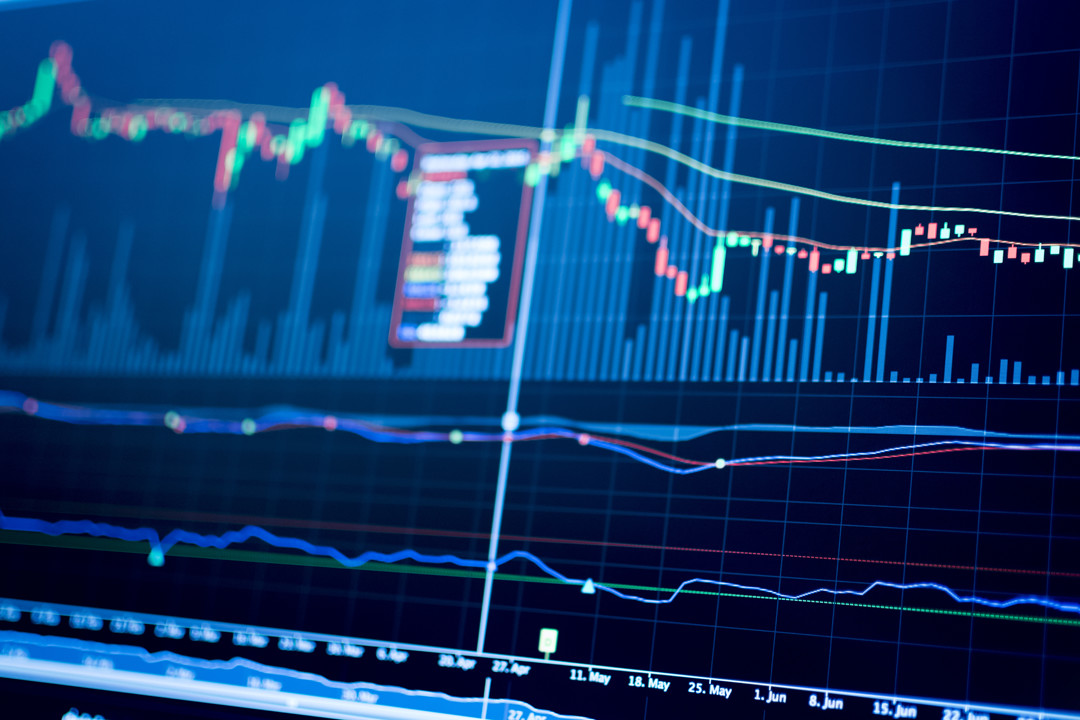# Can entropy be used to forecast risk?Dr Emese Lazar, Dr Alfonso Dufour and Daniel Traian Pele publish new paper 'Information entropy and measures of market risk', which describes how entropy as a financial model can be used to forecast value-at-risk (VaR). Dr Emese Lazar discusses the paper below:

Entropy is a measure of uncertainty. In some ways it is similar to volatility. So, when there is more uncertainty about the financial returns, then entropy increases. It reaches its minimum value when a distribution has no uncertainty (when the outcome is known), and it reaches its maximum value when a distribution is uniform (when all outcomes are equally likely).

Entropy is calculated using the following equation, which uses probabilities only, ignoring the sizes of the possible outcomes:As we depart from normality to distributions with heavier tails (that represent returns with higher tail risk, as in the case of financial returns), the entropy generally decreases.As such, there is a suspected negative relationship between entropy and measures of risk such as Value-at-Risk (VaR) and Expected Shortfall (ES), and we investigate this relationship in our paper. For FX returns, we found evidence of a strong (negative) relationship between entropy and intraday measures of risk. Finally, we used entropy to forecast daily VaR. Our backtest results show that the entropy-based VaR forecasts perform better than competing models.